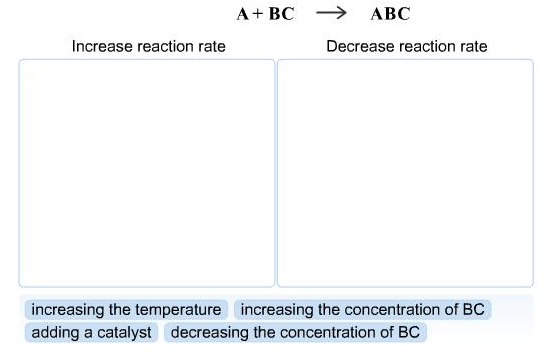# Problem: How would the following events affect the rate of the following general reaction?

🤓 Based on our data, we think this question is relevant for Professor Van Dorn's class at ARIZONA.

###### Problem Details

How would the following events affect the rate of the following general reaction?What scientific concept do you need to know in order to solve this problem?

Our tutors have indicated that to solve this problem you will need to apply the Rate of Reaction concept. You can view video lessons to learn Rate of Reaction. Or if you need more Rate of Reaction practice, you can also practice Rate of Reaction practice problems.

What is the difficulty of this problem?

Our tutors rated the difficulty ofHow would the following events affect the rate of the follow...as medium difficulty.

How long does this problem take to solve?

Our expert Chemistry tutor, Dasha took 3 minutes and 20 seconds to solve this problem. You can follow their steps in the video explanation above.

What professor is this problem relevant for?

Based on our data, we think this problem is relevant for Professor Van Dorn's class at ARIZONA.